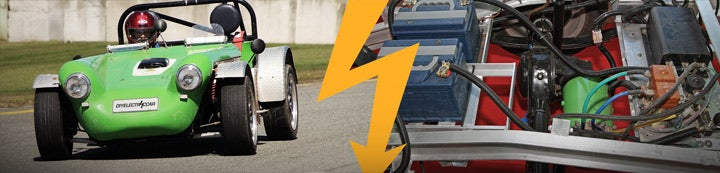1 - 3 of 3 Posts

#### EVDL List

·
##### Registered
Joined
·
70 Posts
Discussion Starter · ·
Hello All

What does Ampere hour signify ?

does a higher ah rating equals more range , what is the ideal Ah
rating for an EV

Thanks
Gulabrao

#### EVDL List

·
##### Registered
Joined
·
70 Posts
Discussion Starter · ·
Hello Gulabrao,

The ampere-hour rating of a battery is the measure on how many amperes it
can draw in a specific amount of time. The standard rating which is choose
by battery companies, is how long it takes to discharge a battery to 0%
State OF Charge (SOC) or is the same as 100% Depth Of Discharge (DOD).

For example:

A battery that can be discharge at 5 amperes at 20 hours, this is a 5 Amps x
20 hrs = 100 AH battery.

A reserved minute rating at 20 hours would be 20 hrs x 60 mins = 1200
reserved minutes.

Now as you increase the ampere draw of the battery, the ampere-hour rating
of the battery decreases. If you increase the the ampere load of the battery
to about 20 amperes, it may only deliver 20 amps for 4 hours, which is a
actual capacity of 80 ampere-hours.

You can convert the reserved minutes that is list for a battery. For
example, a battery with a 100 AH rating with a listed Reserved Minutes of
180 minutes for a 25 amp draw would be about:

180 min / 60 = 3 hrs

3hr x 25 amps = 75 amp-hrs

So you see, when the ampere draw of a battery goes up, you have less
ampere-hour available.

The average ampere draw for a EV is about 75 battery amperes. The reserved
minutes listed for a 100 Ah battery is about 60 minutes at 75 amps which is
about one third the time at 25 reserved minutes.

My Trojan T-145's is a 260 AH at a 20 hr rate battery with a 145 reserved
minutes at 75 amps. The actual ampere-hour would be:

145 mins / 60 = 2.41 hrs

2.41 hrs x 75 amps = 181.25 AH

It is best not to discharge a Pb-Acid battery below 50% DOD, so the maximum
ampere-hour draw out the battery would be 90 AH. For a long life I normally
charge at at 120 ampere-hour remaining in the battery or about 25 DOD%.

Roland

----- Original Message -----
From: "gulabrao ingle" <[email protected]>
To: <[email protected]>
Sent: Monday, July 30, 2007 12:12 AM
Subject: what does Ah or ampere hour signify

> Hello All
>
> What does Ampere hour signify ?
>
> does a higher ah rating equals more range , what is the ideal Ah
> rating for an EV
>
>
> Thanks
> Gulabrao
>
>

#### EVDL List

·
##### Registered
Joined
·
70 Posts
Discussion Starter · ·
Gulabrao Ingle asked:

"What does Ampere hour signify ?

does a higher ah rating equals more range , what is the ideal Ah
rating for an EV?"

**** says: Roland Wiench's separate reply to your question is very accurate.
Here's some more information that may be helpful.

First consider the ampere: that's a measurement for electrical current, but
the amp is a real time value which cannot by itself represent a quantity of
energy (and cannot by itself even represent a quantity of power). The amp is
sort of like "miles per hour" as it is a real time rate of activity.

The amp-hour adds the element of time which takes us closer to measuring a
quantity of energy. The ampere-hour is a product reached when amperage is
multiplied by time. If 100 amperes flow for one hour, that's 100 amp-hours.
If 200 amps flow for a half hour, that also multiplies out to 100 amp-hours.

Battery capacity is often rated by the amp-hour so a battery with more ah of
capacity would also hold more energy, provided that the battery voltage is
the same.

Voltage must enter the picture before one can calculate a quantity of
energy. If the 100 amp-hours described above should move at an assumed 120
volts, multiply these two values together to calculate watt-hours. 100 X 120
= 12,000 watt-hours, which is the same as 12 kilowatt-hours.

I conduct energy calculations mostly in watt-hours instead of in amp-hours,
because the number of watt-hours under discussion always describes the same
amount of energy regardless of the voltage. I find that the watt-hour is
less confusing for my clients than the amp-hour, so I do amperage related
calculations in the background.

Gulabrao asked, "what is the ideal Ah rating for an EV?"

**** says: It's impossible to answer a question like that because you have
not specified the battery voltage, the desired traveling distance, the
weight of the vehicle, or other important factors. Battery system design
includes many parameters which push and pull on one another. The amp-hour is
only one of those factors.

EVDL participants would be well qualified to address some of the other
parameters if they have the time to reply.

**** Abraham, Proprietor
www.abrahamsolar.com

1 - 3 of 3 Posts